# Chlorite ion (ClO2-) lewis dot structure, molecular geometry, hybridization, polarity

Home  > Chemistry Article > ClO2- lewis structure and its molecular geometryChlorite or chlorite ion or chlorine dioxide anion is made up of one chlorine and two oxygen atom with a negative charge having the chemical formula ClO2-. It is also known as salts of chlorous acid. Chlorite is one of the strongest oxidizers of chlorine oxyanions.

In this article, we will study Chlorite ion(ClO2-) lewis structure, molecular geometry, hybridization, polar or nonpolar, bond angle, etc.

Chlorite has a +3 oxidation state and is part of the chlorine oxides family. Chlorite is used in the paper, pulp, and bleaching of textiles.

Properties of Chlorite ion

• It has a molar mass of 67.452 g.
• The conjugate acid of chlorite ion is chlorous acid.
• It is a strong oxidizer.
• It is a salt of chlorous acid.
 Name of Molecule Chlorite ion Chemical formula ClO2- Molecular geometry of ClO2- Bent or V-shaped Electron geometry of ClO2- Tetrahedral Hybridization Sp³ Bond angle Less than 109.5º Total Valence electron for ClO2- 20
Page Contents

## How to draw lewis structure for ClO2-(Chlorite)

ClO2- lewis structure contains one single bond and one double bond. Two lone pairs present on the central atom of the ClO2- Lewis structure. Lewis diagram is a representation of the valence electron within a molecule.

To draw the lewis diagram of any molecule, we have to follow 5 or 6 simple steps depending on the complexity of the molecule.

Here’s in this article, we will draw the ClO2- lewis structure with some easy steps including all explanations.

## Follow some steps for drawing the lewis dot structure of ClO2-

1. Count total valence electron in ClO2-

Counting valence electrons is always the first step we must follow for drawing the lewis diagram of any molecule. To count the valence electron of a molecule, just watch the periodic group number of an atom.

In the case of the ClO2- molecule, chlorine belongs to the 17th periodic group and oxygen 16th. Hence, the valence electron for chlorine is 7 and for oxygen, it is 6.

⇒ Total number of the valence electrons in chlorine = 7

⇒ Total number of the valence electrons in oxygen = 6

∴ Total number of valence electron available for drawing the lewis structure of ClO2- = 7 + 6(2) + 1 = 20 valence electrons         [∴two oxygen atoms, one chlorine and one negative charge that count as a one valence electron]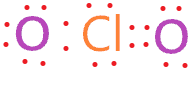2. Find the least electronegative atom and placed it at center

Now we have to find the outer and central atom of the ClO2- molecule. Generally, less electronegative holds the place of the central atom, and the rest are spread evenly around it.

So, from chlorine and oxygen, chlorine(3.16) is less electronegative than oxygen(3.44). Hence, put the chlorine at the center, and oxygen atoms spread around it.3. Connect outer atoms to central atom with a single bond

Here’s in this step, we will connect outer atoms(oxygen) to the central atom(chlorine) with the help of a single bond for further drawing the lewis structure of ClO2-. Just place one-one single bonds between oxygen and chlorine atom.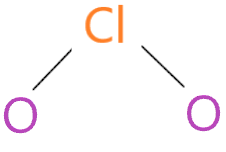After connecting the outer atom to the central atom, count the number of valence electrons we used till now for making the above structure. A single bond formed from two-electron and in the above structure, we used two single bonds.

Hence, 4 valence electrons we have used in the above structure from the 20 available valence electrons for drawing the ClO2- Lewis structure.

(20 – 4) = 16

We have left with 16 valence electrons more.

4. Place remaining valence electrons starting from outer atom first

It’s time to complete the octet of the outer atom first. “The octet rule refers to the tendency of atoms to prefer to have eight electrons in the valence shell”.

So, start putting the remaining valence electrons on the outer atom(oxygen) and complete their octet first.So, we put the 6 valence electron on each oxygen, as oxygen already shares two-electron with the help of a single bond. Each oxygen in the above structure shares 8 electrons, hence completed their octet comfortably.

As we had a total of 16 valence electrons and in the above structure, we used 12 more electrons.

(16 – 12) = 4 valence electrons

Now we are still left with 4 more valence electrons.

5. Complete central atom octet and make covalent bond if necessary

In ClO2- molecule, chlorine is the central atom and it needs 8 electrons around to complete the octet. As you see in the structure of the 4th step, chlorine already sharing 4 electrons with the help of two single bonds connected to oxygen atoms.

So, just put the 4 leftover valence electrons on chlorine and complete its octet.By looking at the above structure, we see both atoms(oxygen and chlorine) in ClO2- molecule completed their octet comfortably and we used all our 20 total valence electrons available for drawing the ClO2- Lewis structure.

But we don’t know if the above ClO2- Lewis structure is stable or not, so we can’t assume the above structure, as the best and stable lewis structure of ClO2-.

For checking the stability of the lewis diagram, we will go through the concept of a formal charge.

6. Check the stability with the help of a formal charge concept

“A formal charge is a charge assigned to an atom in a molecule, assuming that electrons in all chemical bonds are shared equally between atoms.”

The structure with the formal charge close to zero or zero is the best and stable lewis structure.

We will calculate the formal charge on the 5th step structure.

⇒ Formal charge = (valence electrons – lone pair electrons –  1/2bonded pair electrons)

For chlorine atom –

⇒ Valence electron of chlorine = 7

⇒ Lone pair electrons on chlorine = 4

⇒ Bonded pair electrons around chlorine = 4

F.C. on chlorine atom = (7 – 4 – 4/2) = +1

For oxygen atom –

⇒ Valence electron of oxygen = 6

⇒ Lone pair electrons on oxygen = 6

⇒ Bonded pair electrons around oxygen = 2

F.C. on oxygen atom = (6 – 6 – 2/2) = -1The above structure is not much stable, as the lewis structure is the most stable when the formal charge on each atom and an overall formal charge of the molecule is close to zero or zero.

Let’s do some twists on the above structure to get the formal charge close to zero.

As we know, chlorine can hold more than 8 electrons because it has d orbital for extra electrons needed for bonding.[Exception to the octet rule

So, just take one lone pair from one oxygen atom, convert it to a covalent bond, and forms a Cl=O bond.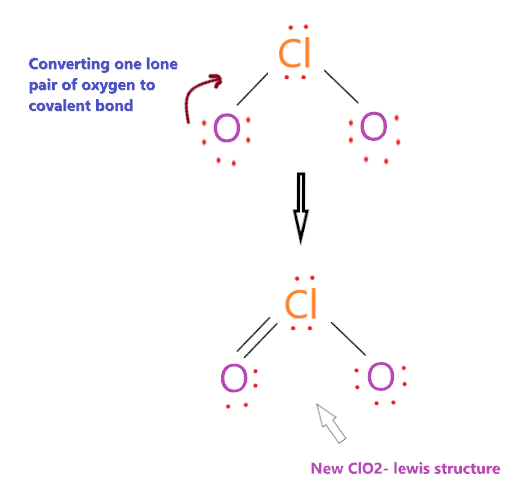Now again determine the formal charge on the new ClO2- Lewis structure.

For chlorine atom –

⇒ Valence electron of chlorine = 7

⇒ Lone pair electrons on chlorine = 4

⇒ Bonded pair electrons around chlorine = 6

F.C. on chlorine atom = (7 – 4 – 6/2) = 0

For oxygen atom(left side) –

⇒ Valence electron of oxygen = 6

⇒ Lone pair electrons on oxygen = 4

⇒ Bonded pair electrons around oxygen = 4

F.C. on oxygen atom = (6 – 4 – 4/2) = 0

For oxygen(right side) –

⇒ Valence electron = 6

⇒ Lone pair electrons on oxygen = 6

⇒ Bonded pair electrons around oxygen = 2

F.C. on oxygen atom = (6 – 6 – 2/2) = -1

∴ We get (-1) overall formal charge on the new ClO2- lewis structure and two atoms have zero formal charges, hence, this lewis structure of ClO2- is stable and better than the previous structure(5th step structure).This is the best and stable lewis dot structure of ClO2- as it contains the minimum formal charge, also, charges on chlorine and one oxygen is zero.

ClO2- molecule contains a negative charge also, so, we put -1 outside the bracket in the above structure. Also, Chlorite ions can exist in multiple resonant states due to the shifting of bonds.

## What is the molecular geometry of ClO2-?

“Molecular geometry is the three-dimensional arrangement of atoms in a molecule”. The molecular geometry of ClO2- is bent or V-shaped.

According to the lewis structure of ClO2-, chlorine is the central atom that has 2 lone pairs on it, these lone pairs occupy more space than bond pair electrons and try to repel each other. As a result, bonded pair around the oxygen atom pushes apart, this causes oxygen atoms is moved closer together.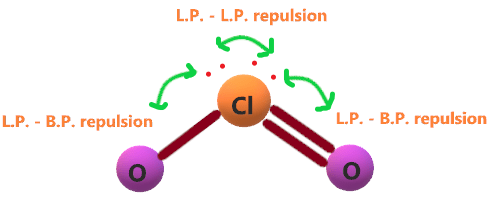Therefore, the resultant molecular geometry of ClO2- appears like a bent or V-shaped.

Let’s see how to find the molecular geometry of ClO2- using the AXN method and VSEPR theory.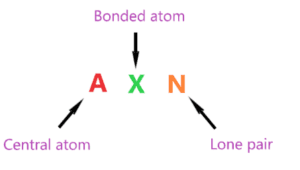That’s how the AXN notation follows as shown in the above picture.

AXN notation for ClO2- molecule:

• A represents the central atom, so as per the ClO2- lewis structure, chlorine is the central atom. A = Chlorine
• X represents the bonded atoms, as we know, chlorine is attached to two oxygen atoms. Therefore, X = 2
• N represents the lone pair, the chlorine atom has two lone pairs on it. Hence, N = 2

So, the AXN formula for the ClO2- molecule becomes AX2N2.

According to the VSEPR theory, if a molecule central atom is attached with two bonded atoms and has two lone pairs then the molecular geometry of that molecule is V-shaped or bent.

Hence, the molecular shape or geometry for ClO2- is bent.### ClO2- molecular geometry

The electron geometry for ClO2- is tetrahedral.## Hybridization of ClO2-

To find the hybridization of an atom, we have to first determine its hybridization number.

“Hybridization number is the addition of a total number of bonded atoms around a central atom and the lone pair present on it.”

∴ Hybridization number of ClO2- = (Number of bonded atoms attached to chlorine + Lone pair on chlorine)

According to the lewis structure of ClO2-, we have two bonded atoms(oxygen) attached to the chlorine and two lone pair present on it.

∴ Hybridization number of ClO2- = (2 + 2) = 4

 Hybridization number Hybridization 1 S 2 Sp 3 Sp² 4 Sp³ 5 Sp³d 6 Sp³d²

So, for a hybridization number of four, we get the Sp3 hybridization on the chlorine atom in the ClO2- molecule.

## The bond angle of ClO2-

“A bond angle is the geometrical angle between two adjacent bonds”.

The bond angle of ClO2- is less than 109° due to the presence of two lone pairs on chlorine atoms as these lone pairs repel each other and that pushes bonded atoms closer together, hence causes the lower bond angle.## Chlorite ion polarity: is ClO2- polar or nonpolar?

Definitely ClO2- is polar in nature as it lacks symmetry because it has a molecular geometry of bent, which means dipole generated along with Cl-O bond unable to canceled out each other giving some dipole moment in the molecule.

The lone pair presence on chlorine causes an unsymmetrical charge distribution in the molecule, so the induced charges on the Cl-O bond do not cancel each other completely.

Also, the presence of a negative charge over the atoms makes the Cl-O bonds polar in nature.

Hence, all these factors lead to ClO2- a polar molecule in nature.

## FAQ

### Why does the chlorine in ClO2- lewis structure, contains electrons of more than 8 and violate the octet rule?

Whenever the d-orbitals and beyond to it, participate in bonding with other atoms then an expanded octet is produced.

Chlorine atom in ClO2- lewis structure expanded the octet because it has d-orbitals in the third principal energy level, hence, it has extra orbital(d-orbital) for additional electron needed for bonding.

Sulfur, phosphorous, and silicon are some other examples that can expand their octet and hold electrons more than 8.

### Why does the molecular geometry of ClO2- appears bent in shape?

This is because of the presence of two lone pairs on chlorine central atom that pushes both oxygen atoms closer together, this causes a lower O-Cl-O bond angle, hence, its shape appears as bent like structure.

## Summary

• The total valence electron available for the Chlorite (ClO2-) lewis structure is 20.
• The hybridization of chlorine in the ClO2- the molecule is Sp3.
• ClO2- is a polar molecule due to the asymmetrical distribution of charges caused by the presence of lone pair electrons.
• The overall formal charge in ClO2- is -1.
• The bond angle in ClO2- is slightly less than 109°.
• The molecular geometry of ClO2- is V-shaped and electron geometry is tetrahedral.
• Total number of 7 lone pairs and 3 bond pairs present in the lewis dot structure of ClO2-
##### Subscribe to Blog via Email

Join 2 other subscribers

Share it...